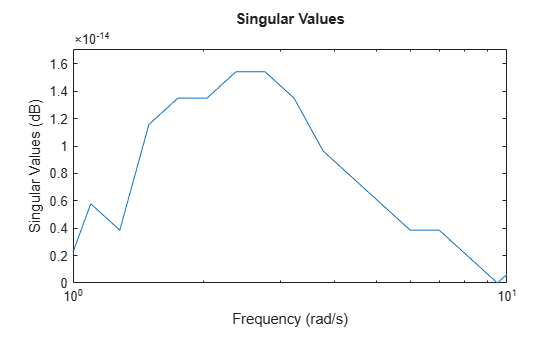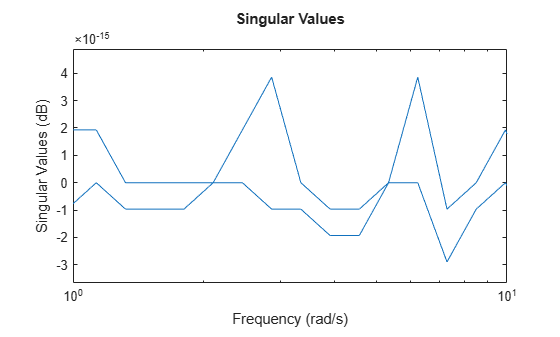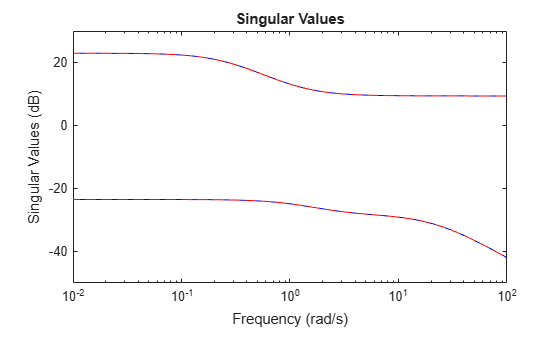Documentation

rncf

Right normalized coprime factorization

Description

example

fact = rncf(sys) computes the right normalized coprime factorization of the dynamic system model sys. The factorization is given by:

$sys={N}_{r}{M}_{r}^{-1},\text{ }{M}_{r}^{*}{M}_{r}+{N}_{r}^{*}{N}_{r}=I.$

Here, ${M}_{r}^{*}$ denotes the conjugate of Mr (see ctranspose). The returned model fact is a minimal state-space realization of the stable system [Mr;Nr]. This factorization is used in other normalized coprime factor computations such as model reduction (ncfmr) and controller synthesis (ncfsyn).

example

[fact,Mr,Nr] = rncf(sys) also returns the coprime factors Mr and Nr.

Examples

collapse all

Compute the right normalized coprime factorization of a SISO system.

sys = zpk([1 -1+2i -1-2i],[-1 2+1i 2-1i],1);
[fact,Mr,Nr] = rncf(sys);

Examine the original system and its factors.

sys
sys =

(s-1) (s^2 + 2s + 5)
--------------------
(s+1) (s^2 - 4s + 5)

Continuous-time zero/pole/gain model.
zpk(Mr)
ans =

0.70711 (s+1) (s^2 - 4s + 5)
----------------------------
(s+1) (s^2 + 3.162s + 5)

Continuous-time zero/pole/gain model.
zpk(Nr)
ans =

0.70711 (s-1) (s^2 + 2s + 5)
----------------------------
(s+1) (s^2 + 3.162s + 5)

Continuous-time zero/pole/gain model.

The numerators of the factors Mr and Nr are the denominator and numerator of sys, respectively. Thus, sys = Nr/Mr. rncf chooses the denominators of the factors such that the system $\left[{\mathit{M}}_{\mathit{r}}\left(\mathit{j}\omega \right);{\mathit{N}}_{\mathit{r}}\left(\mathit{j}\omega \right)\right]$ is a unit vector at all frequencies. To confirm that property of the factorization, examine the singular values of fact, which is a stable minimal realization of $\left[{\mathit{M}}_{\mathit{r}}\left(\mathit{j}\omega \right);{\mathit{N}}_{\mathit{r}}\left(\mathit{j}\omega \right)\right]$.

sigma(fact)Within a small numerical error, the singular value of fact is 1 (0 dB) at all frequencies.

Compute the right normalized coprime factorization of a state-space model that has two outputs, two inputs, and three states.

rng(0); % for reproducibility
[fact,Mr,Nr] = rncf(sys);

fact is a stable minimal realization of the factorization given by [Mr;Nr].

isstable(fact)
ans = logical
1

Another property of fact is that its frequency response F(jω) is an orthogonal matrix at all frequencies (F(jω)'F(jω) = I). Confirm this property by examining the singular values of fact. Within a small numerical error, the singular values are 1 (0 dB) at all frequencies.

sigma(fact)Confirm that the factors satisfy sys = Nr/Mr by examining the singular values of both.

sigma(sys,'b-',Nr/Mr,'r--')Input Arguments

collapse all

Input system to factorize, specified as a dynamic system model such as a state-space (ss) model. If sys is a generalized state-space model with uncertain or tunable control design blocks, then the function uses the nominal or current value of those elements. sys cannot be an frd model or a model with time delays.

Output Arguments

collapse all

Minimal realization of [Mr;Nr], returned as a state-space model. fact is stable and its frequency response is an orthogonal matrix at all frequencies. If sys has p outputs and m inputs, then fact has m+p outputs and m inputs. fact has the same number of states as sys.

Right coprime factors of sys, returned as state-space models. If sys has p outputs and m inputs, then:

• Mr has m outputs and m inputs.

• Nr has p outputs and m inputs.

Both factors have the same number of states as sys and the same A and B matrices as fact.

Tips

• fact is a minimal realization of [Mr;Nr]. If you need to use [Mr;Nr] or [Mr;Nr]' in a computation, it is better to use fact than to concatenate the factors yourself. Such manual concatenation results in extra (nonminimal) states, which can lead to decreased numerical accuracy.